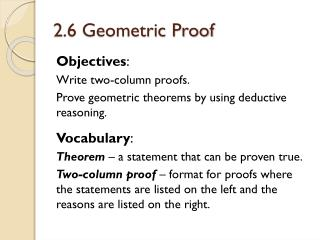# 2.6 Geometric Proof - PowerPoint PPT PresentationDownload Presentation2.6 Geometric Proof

2.6 Geometric Proof
Download Presentation## 2.6 Geometric Proof

- - - - - - - - - - - - - - - - - - - - - - - - - - - E N D - - - - - - - - - - - - - - - - - - - - - - - - - - -
##### Presentation Transcript

1. 2.6 Geometric Proof Objectives: Write two-column proofs. Prove geometric theorems by using deductive reasoning. Vocabulary: Theorem – a statement that can be proven true. Two-column proof – format for proofs where the statements are listed on the left and the reasons are listed on the right.

2. Definitions • Postulates • Properties • Theorems Conclusion Hypothesis 2.6 Geometric Proof When writing a proof, it is important to justify each logical step with a reason. You can use symbols and abbreviations, but they must be clear enough so that anyone who reads your proof will understand them.

3. 2.6 Theorems

4. 2.6 Theorems

5. 2.6 Writing a Proof A geometric proof begins with Given and Prove statements, which restate the hypothesis and conclusion of the conjecture. In a two-column proof, you list the steps of the proof in the left column. You write the matching reason for each step in the right column. Before you start writing a proof, you should plan out your logic. Sometimes you will be given a plan for a more challenging proof. This plan will detail the major steps of the proof for you.

6. Helpful Hint If a diagram for a proof is not provided, draw your own and mark the given information on it. But do not mark the information in the Prove statement on it. 2.6 Tips

7. 2.6 Practice Write the letter of the correct justification next to each step. (Use one of them twice) B A B C

8. 2.6 Proof Statements 1. 2. 3. 4. Reasons 1. Given 2. 3. Substitution Prop. of = 4.

9. 2.6 ProofMark the diagram and answer the questions about the proof on the following slide. Statements Reasons

10. 2.6 Proof Questions • What was the given information? • What should be marked in the diagram? • What was the conjecture to be proved? • What titles should be put above the two columns?

11. 2.6 Video explanation for Proofs Copy the link below and paste it into the address bar to visit the website. Watch the video explanations for the sample proof problems. Link to Holt textbook video lessons: http://my.hrw.com/math06_07/nsmedia/homework_help/geo/geo_ch02_06_homeworkhelp.html